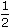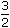# Aptitude - Banker's Discount - Discussion

Discussion Forum : Banker's Discount - General Questions (Q.No. 4)
4.
The banker's discount on a sum of money for 1years is Rs. 558 and the true discount on the same sum for 2 years is Rs. 600. The rate percent is:
10%
13%
12%
15%
Explanation:

B.D. foryears = Rs. 558.
B.D. for 2 years
 = Rs.558 x 2 x 23
= Rs. 744

T.D. for 2 years = Rs. 600.Sum = B.D. x T.D. = Rs.744 x 600= Rs. 3100. B.D. - T.D 144

Thus, Rs. 744 is S.I. on Rs. 3100 for 2 years.Rate =100 x 744% = 12% 3100 x 2

Discussion:
21 comments Page 1 of 3.

Rohit said:   1 year ago
FOR TWO YEARS THE BD IS:

588 * (2/3) * 2 = 784. (not 744).

The final answer comes out to be 16.6667.

Bhanu said:   3 years ago
Thanks @Ritu.

Ankur Singh said:   4 years ago
let the present worth be x then BD for 18 months will be;

x(1+3R/100)*3R/100= 558------------->(i)
TD for 2 years.

xR*2/100 = 600----------------> (ii)
Dividing (i)/(ii).

Will get R = 16.
(1)

Likitha said:   4 years ago
How bankers discount for 2 yrs= 558*2/ (3/2) came?
(1)

Kathir said:   5 years ago
For 2 years X = 558 * 2.

Divya said:   5 years ago
How come X=558*2?

Ritu said:   5 years ago
B.D = A*r*t1/100 ---> (1) and
T.D. = A*r*t2/(100+rt2) ---> (2).

Now as the amount or sum of money is same so from (1) A= B.D*100/(r*t1).
From (2) A= T.D*(100+r*t2)/(r*t2).
Put values and compare both as 'A' is same.
558*100/(r*3/2) = 600*(100+2r)/(2r).
55800*2/3 = 300(100+2r).

On solving
1860 = 1500 + 30r.
r = 360/30.
(1)

Kamal said:   5 years ago
What is true dicount?

Megha Mahale said:   5 years ago
What is the Formula for the rate?

Magesh said:   6 years ago
I can't understand the solution can anyone help me to understand in simple way?